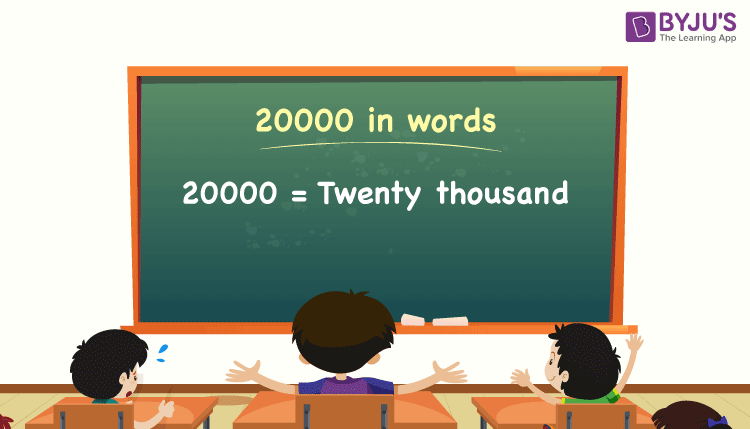# 20000 in Words

20000 in words can be written as Twenty Thousand. If you paid a 20000 bill for purchasing a TV at the showroom, you could say that “I paid twenty thousand rupees” for this TV. As we know, 20000 is a cardinal number since it denotes a specific quantity.

 20000 in words: Twenty thousand Twenty thousand in numerical form: 20000

20000 in English words

We generally write numbers in words using the English alphabet. Thus, we can read 20000 using the words in English as “Twenty Thousand”.## How to Write 20000 in Words?

We can convert 20000 to words using a place value chart. This can be done as follows.

The number 20000 has 5 digits, so let’s make a chart that shows the place value up to 5 digits.

 Ten thousand Thousands Hundreds Tens Ones 2 0 0 0 0

Thus, we can write the expanded form as:

2 × Ten thousand + 0 × Thousand + 0 × Hundred + 0 × Ten + 0 × One

= 2 × 10000 + 0 × 1000 + 0 × 100 + 0 × 10 + 0 × 1

= 20000

= Twenty thousand

Alternative method:

In the above place value chart, we can observe that the digits in ones, tens, hundreds and thousands are 0s. Hence, we can consider the number at the ten thousand place, and by combining these terms, we get twenty thousand since 2 × 10 = 20, i.e. multiplying ten by 2, we get twenty.

Interesting way of writing 20000 in words:

2 = Two

20 = Twenty

200 = Two hundred

2000 = Two thousand

20000 = Twenty thousand

20000 is the natural number that is succeeded by 19999 and preceded by 20001.

20000 in words – Twenty thousand

Is 20000 an odd number? – No

Is 20000 an even number? – Yes

Is 20000 a perfect square number? – No

Is 20000 a perfect cube number? – No

Is 20000 a prime number? – No

Is 20000 a composite number? – Yes

## Frequently Asked Questions on 20000 in Words

Q1

### What is the correct spelling of 20000?

The spelling of 20000 in English words is Twenty thousand, that means 20000 in words can be pronounced as twenty thousand.

Q2

### How do you write 20000 rupees in words?

20000 rupees in words can be written as Twenty thousand rupees.

Q3

### How do you write 20000 in words for a cheque?

For a cheque, we generally write 20000 in words as Twenty thousand rupees only.

Q4

### Write 22000 in words.

22000 in words will be Twenty-two thousand, i.e. 22 × 1000 = 22000 = twenty-two thousand.